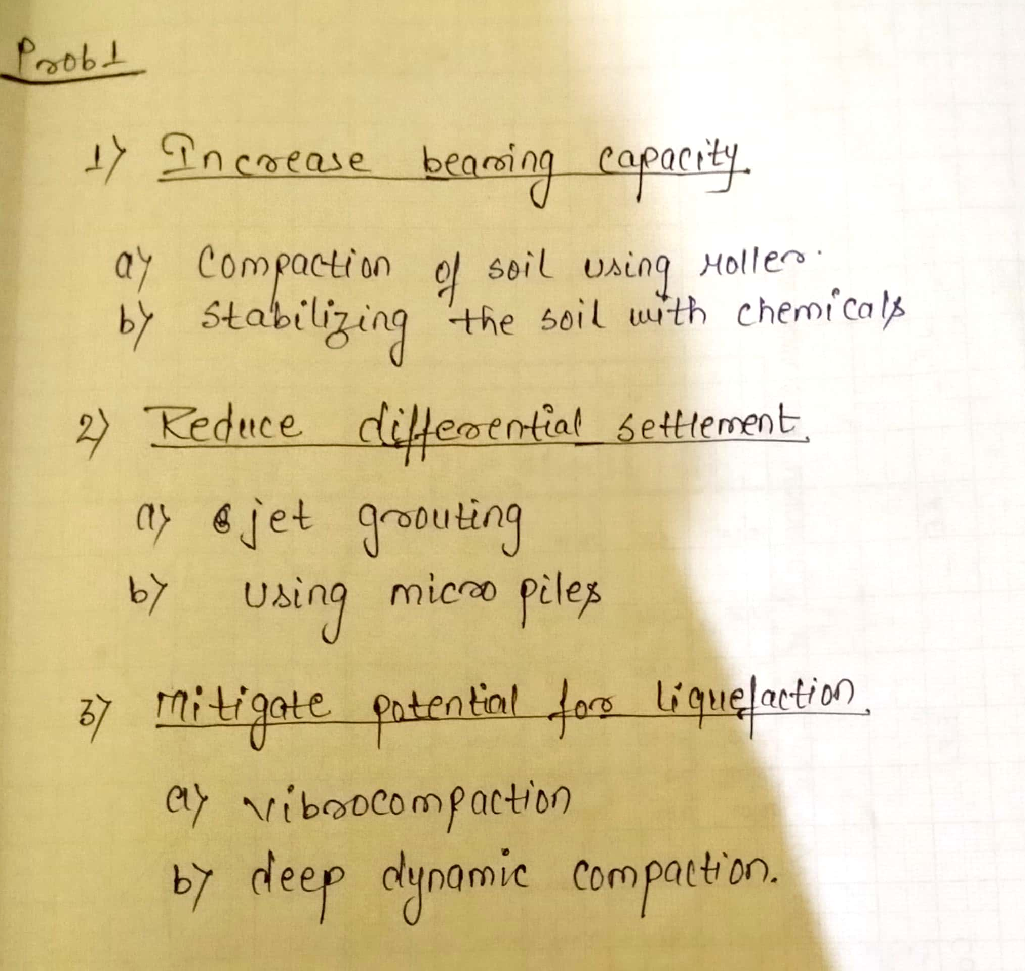Question

Soil Mechanics

We've discussed a variety of ground improvement strategies in class so far. Use your knowledge of the ground improvement techniques that we've discussed in class to suggest at least two ground improvement strategies for optimizing the following engineering properties. Be specific about which technique you recommend(i.e. which type of compaction? should I include vertical drains?)

1. Increase bearing capacity

2. Reduce differential settlement

3. Mitigate potential for liquefactionVerified### Question 36963Soil Mechanics

e Consolidation test on a representative sample of the normally consolidated clay soil stratum, shown in the soil profile below, resulted in a compression index of0.28. A structure proposed at this site will produce a uniform surface load of 100kPa on a mat foundation (10 m by 15 m) at the ground surface. Estimate the maximum potential differential settlement throughout the foundation.

### Question 36962Soil Mechanics

A 6m thick saturated compressible clay stratum has an average overburden effective stress of 120 kpa and a void ratio of 0.96 construction loads at this site will result in a stress increase of approximately 180kpa in the middle of the clay layer construction test on a representative sample of the clay soil resulted in the following compression data

### Question 36961Soil Mechanics

a consolidation test on a representative sample of soil obtained from the middle of the clay layer shown in the soil profile resulted in the following laboratory compression curve. reconstruct and plot the field compression curve show all the steps

### Question 36960Soil Mechanics

why may lowering groundwater table induce settlement

### Question 36959Soil Mechanics

what is an overconsolidated clay and what causes the overconsolidation give three possible causes.

### Question 33710Soil Mechanics

\text { 7. Given } f_{c}^{\prime}=5,000 p s i \text { (Normal Weight Concrete, } 145 \text { lbs } \sqrt{f}^{3} \text { ). }
(a) Follow the ACI code to estimate f' (modulus of rupture) and E. (modulus of elasticity)for the concrete beam shown above.

### Question 33709Soil Mechanics

6. A mixture of 1080 g of gravel with an effective absorption of 0.90% and 720 g of sand with a surface moisture of 2.51% was added to a concrete mix. Compute the adjustment that must be made to the added water to maintain a constant w/c ratio.

### Question 33708Soil Mechanics

5. A 1000-g sample of sand from the same stockpile as in Problem 3 weighted 972 g when dried at 105°C overnight. Calculate the surface moisture of the sand. (Compare your answer with the answer to Problem 3).

### Question 33707Soil Mechanics

4. The sample of sand in Problem 2 weighted 495 g after drying at 105 °C overnight. Calculate theabsorption capacity of the sand.

### Question 33706Soil Mechanics

3. A 500-g sample of the same sand as in Problem 2 from the stockpile weighted 1691gwhen placed in the jar and topped up with water. Calculate the moisture content of the sand in the stockpile.

### Submit query